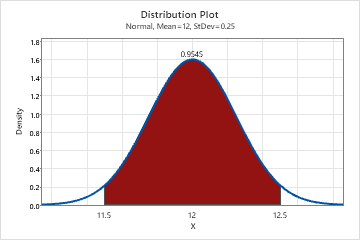# Example of a probability distribution plot with a shaded region

An engineer for a soda bottling facility collects data on soda can fill weights. The engineer determines that the fill weights follow a normal distribution with a mean of 12 ounces and a standard deviation of 0.25 ounces. The engineer analyzes the distribution of the data to determine the probability that a randomly chosen can of soda has a fill weight that is between 11.5 and 12.5 ounces.

1. Choose Graph > Probability Distribution Plot.
2. Select View Probability, then click OK.
3. From Distribution, select Normal.
4. In Mean, enter 12.
5. In Standard deviation, enter 0.25.
6. Click the Shaded Area tab.
7. In Define Shaded Area By, select X value.
8. Click the Middle icon. This option shows the probability that is between two x-values.
9. In X value 1, enter 11.5. In X value 2, enter 12.5.
10. Click OK.

## Interpret the results

If the population of fill weights follows a normal distribution and has a mean of 12 and a standard deviation of 0.25, then the probability that a randomly chosen can of soda has a fill weight that is between 11.5 and 12.5 ounces is 0.9545.By using this site you agree to the use of cookies for analytics and personalized content.  Read our policy